Importance: Medium ✭✭
 Author(s): Steffen, Eckhard
 Subject: Graph Theory » Coloring » » Nowhere-zero flows
 Keywords: nowhere-zero flow, edge-colorings, regular graphs
 Posted by: Eckhard Steffen on: August 5th, 2015

A nowhere-zero-flowonis an orientation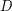oftogether with a functionfrom the edge set ofinto the real numbers such that, for all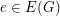, and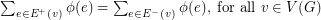. The circular flow number ofis inf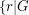has a nowhere-zero-flow, and it is denoted by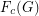.

A graph with maximum vertex degreeis a class 1 graph if its edge chromatic number is.

Conjecture   Letbe an integer anda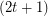-regular graph. Ifis a class 1 graph, then.

The conjecture is true for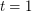, i.e. for cubic graphs. It says, that the circular flow number of-regular class 1 graphs is bounded by the circular flow number of the complete graph on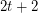vertices.

## Bibliography

[ES_2001] E. Steffen, Circular flow numbers of regular multigraphs, J. Graph Theory 36, 24 – 34 (2001)

*[ES_2015] E. Steffen, Edge-colorings and circular flow numbers on regular graphs, J. Graph Theory 79, 1–7, 2015

* indicates original appearance(s) of problem.